Study, analysis and simulation of a static compensator D-STATCOM for distribution systems of electric power

Ahmed MOKHTARI1*, Fatima Z. GHERBI1, Cherif MOKHTAR1, Kamel D. E. KERROUCHE2, Ameur F. AIMER3

1Intelligent Control and Electrical Power Systems Laboratory ICEPS Department of Electrical Engineering, Djillali Liabes University of Sidi Bel Abbes, Algeria.

2Electrical Engineering Department, Dr. MOULAY TAHAR University, Saida, Algeria

3Diagnosis Group-LDEE Laboratory, University of Science and Technology of Oran, Algeria.

E-mail: mokhtari07_usto@yahoo.fr

Corresponding author, Phone: +213 48 50 24 78

Abstract

Flexible Alternative Current Transmission System « FACTS » devices are controllers based on power electronics. It has been demonstrated that they can improve transient stability and help to damp electromechanical oscillations in distribution power systems. The aim of this paper was to clarify the power flow without and with a Distribution Static Compensator « D-STATCOM » and to improve the quality of the Algerian network. It is a shunt compensation device which is generally used to solve power quality problems in distribution systems. The present work will use these devices to study the functions available in the control of reactive power compensation as well as maintaining voltage.  The simulation is done under MATLAB/SIMULINK for D-STATCOM and voltage source converter (VSC). Through this paper, D-STATCOM has demonstrated excellent performance and effectively improved the power quality in distribution networks.

Keywords

Flexible alternative current transmission system (FACTS); Distribution networks; Compensation; D-STATCOM; Voltage source converter (VSC)

Introduction

Conventional compensation systems, such as capacitor banks, inductance mechanically driven and synchronous machines, have been used for long to increase the power flow in steady state by controlling the voltage of the distribution network .

It has been shown that transient stability as well as stability in permanent system of an electrical network can be improved if the compensation can react quickly using thyristors as switches and in particular the new controllable components in both opening and closing (GTO, IGBT) . To avoid losses by Joule effect on distribution lines caused by inductive currents (mainly caused by the consumer but also by the line itself) and also avoid the same voltage drops in result, we should compensate the reactive power. As already mentioned, the compensation of the reactive power can be achieved in several ways. Most of these compensators are efficient but still have some defects: high reaction time or harmonic generation.

In electrical distribution there are two main families of applications based on three-phase voltage inverter structure . On the other hand, the systems called « D-FACTS » (Flexible AC Transmission System dedicated to the distribution network) and secondly, interfaces to connect to the network of decentralized energy producers.

Systems based on electronic power can be connected to the network in series compensator like DVR (Dynamic Voltage Restorer) or shunt compensator like D-STATCOM (static compensator distribution), and can use a mode of active or passive compensation. Depending on their mode of connection, they behave as current sources (case shunt), or as voltage sources (case series) [4, 5]. The inverter voltage associated with the shunt connection is by abuse of language called D-STATCOM (D as Distribution and STATCOM for STATic Compensator). The abuse language comes owing to the fact that the assured functions largely exceed the adjustment of reagent, maintaining a voltage level of reactive power exchange with the network and sometimes for some vibration damping.

The study presented in this paper is based on the control voltage for a shunt compensation of reactive power with a FACTS device based voltage inverter D-STATCOM. A complete simulation of DSTATCOM is carried out in MATLAB/SIMULINK, introduced into the distribution network is presented to validate the operation of this device in both modes of capacitive and inductive compensation.

In addition, this work is based on the simulation and realization of a D-STATCOM for the compensation of the load in a distribution network of electrical energy.

Material and method

Description

In 1990 the first STATCOM has been designed, it is a voltage converter based GTO or IGBT powered by batteries capacitor, which are coupled in parallel to the system through a coupling transformer Figure 1 (a). This device is similar to a synchronous compensator, and as it is an electronic device, it has no mechanical inertia while having improved better characteristics such as its fast dynamics and low cost of installation and its maintenance to synchronous condensers .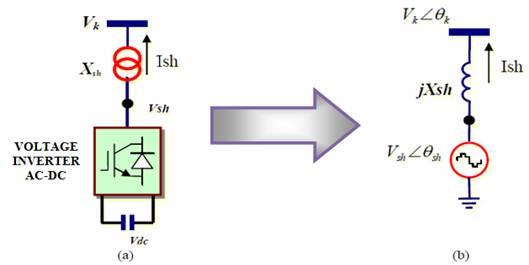Figure 1. (a) Structure of a D-STATCOM, (b) Equivalent circuit

Definition According to IEEE 

D-STATCOM is a static synchronous generator operating as a Static Var Compensator (SVC) connected in parallel with the output current (capacitive or inductive) can be controlled independently of the AC voltage network.

The principal functions of D-STATCOM

• Mitigate the impact of dips and peaks of voltage sensitive loads.

• Voltage regulation.

• Compensation of harmonic currents.

• Control of the reactive power.

• Mitigation of Voltage Flicker.

• Uninterrupted supply in case of use of an energy storage device.

Principle of D-STATCOM

The shunt compensators are well recognized in the compensation of reactive power and therefore the regulation of voltage bus bar where they are connected. The D-STATCOM is a static synchronous generator that generates a synchronous three-phase alternative voltage with the mains voltage from a direct voltage source. The amplitude of the voltage of D-STATCOM may be controlled to adjust the quantity of reactive power to share with the network .

In general, the voltage of D-STATCOM Vsh is injected in phase with the line voltage Vt, and in this case there is no exchange of energy with the active network, but only reactive power to be injected (or absorbed) by the D-STATCOM as summarized in Figure 2.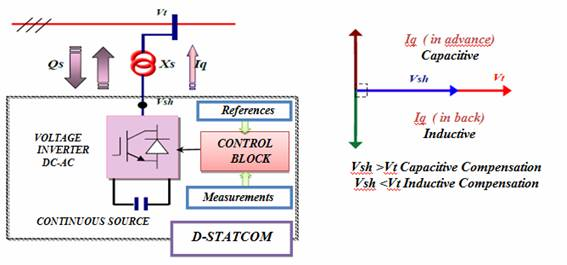Figure 2. The basic structure of a D-STATCOM coupled to the network

The reactive power exchange with the network is done by varying the amplitude of the output voltages.

Depending on the amplitude of these tensions, the three following operating systems are available for the D-STATCOM. The basic principle of operation for a D-STATCOM is explained with the help of Figure 2. The output voltage of the GTO converter (Vsh) is controlled in phase with the system voltage (Vt), as shown in this figure, and the output current of the D-STATCOM (Iq) varies depending on Vsh, [8, 9]. If:

1. Vt<Vsh: The phase angle of Iq is leading with respect to the phase angle of Vt by 90 degrees. As a result, leading reactive power flows from the D-STATCOM (capacitive mode).

2. Vt>Vsh: The phase angle of Iq is lagging with respect to Vt by 90 degrees; the D-STATCOM consumes reactive power flows and then an inductive mode.

3. Vt = Vsh: Then no reactive power is delivered to the power system.

As a result, lagging reactive power flows into the STATCOM (inductive mode). The amount of the reactive power is proportional to the voltage difference between Vt and Vsh. Note that this is the same basic operating principal as a rotating synchronous condenser .

The variation of the output voltages amplitude is achieved by varying the direct voltage across the capacitor. The D-STATCOM can deliver a capacitive or inductive current independent of the network voltage. So it can provide the maximum capacitive current even at low voltage values. Its ability to support the supply voltage is better than the SVC. Moreover, the D-STATCOM can temporarily increase the nominal current (inductive or capacitive).

The advantage of this device is able to exchange energy nature (capacitive or inductive) only with an inductor. Unlike SVC, there is no capacitive element that can cause resonances with inductive elements of the network. The structure and operational characteristic is shown in Figure 3. The STATCOM smoothly and continuously controls voltage from V1 to V2. However, if the system voltage exceeds a low-voltage (V1) or high-voltage limit (V2), the STATCOM acts as a constant current source by controlling the converter voltage (Vi) appropriately .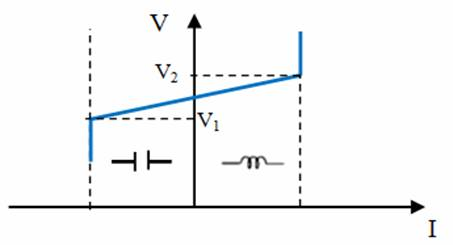Figure 3. V- I Characteristic of a STATCOM.

Mathematical formulation of D-STATCOM

There are three modelling approaches, average modelling, detailed modelling and phase to phase modelling. Generally phase to phase modelling is not used for D-STATCOM [11, 12]. Mathematical models of voltage converters for various electrical connections in a serial or parallel network are very useful.

Considering the D-STATCOM in Figure 2, the equivalent circuit of this device with the energy system (voltage source, line and load) is given in Figure 4.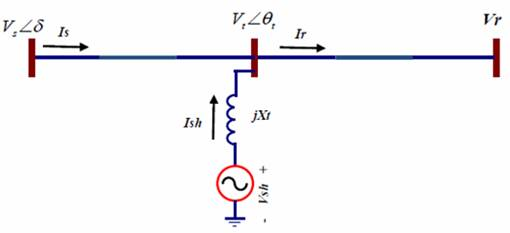Figure 4. Equivalent circuit of the D-STATCOM connected to a network.

By making the voltage connection point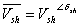as reference phase and the fundamental component of the Voltage Source Converter (VSC) is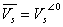. The active and the reactive power exchanged with network are given by the equations:(1)

The STATCOM, as it was already described in Figure 4, can be represented by the equivalent circuit taking into account only busbar where this device is connected.

The current injected by the STATCOM is given by: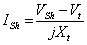(2)

where quantities are in three phase:(3)

The power injected to the bus bar is given by the following equation: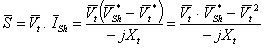(4)

Where it led to active and reactive power injected by the STATCOM to the bus bar expressed by the following formulas: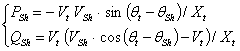(5)

To study the relationship between the grid and the STATCOM we will adopt it for the mathematical model of Figure 4, he current of the STATCOM depends on the difference between the system voltage Vt (voltage at node) and adjustable voltage STATCOM. We applying Ohm's law we have: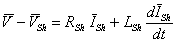(6)

We turns rotating frame (d,q), the repository synchronization, multiplying all quantities by phasor e- jg  with dg/dt = w the pulse of the quantities concerned.

Therefore: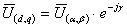and conversely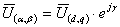Applying this writing on the equation (6) we have: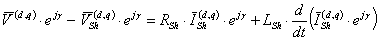(7)

After simplification of calculation leads to dynamic equations of STATCOM in the frame (d,q) as follows:(8.a)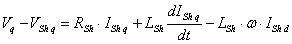(8.b)

In matrix form, we write the system state of the STATCOM as follows:(9)

From equation (9), the block diagram of the STATCOM main system in d-q frame is established and depicted in Figure 5.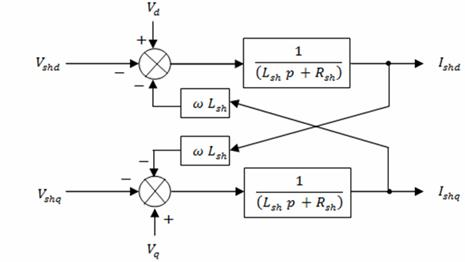Figure 5. The coupled STATCOM model.

Control of D-STATCOM

Classical PI control is the most common industrial used technique. This is due to recess the simplicity of its implementation, its acceptable performance. This is achieved by a judicious choice of parameters "Kp, Ki", representing the proportional gain and the gain of the integral action. Figure 6 shows the configuration used with this type of adjustment for the control of D-STATCOM.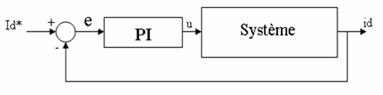Regulation parameters "Kp, Ki" are obtained by the method of pole placement.

Results and discussion

In this work, detailed simulations are performed on the D-STATCOM equivalent circuit connected to an industrial system, which was modelled using MATLAB/SIMULINK to observe the behaviour of the network with different types of disturbances as shown in Figure 7, System parameters are listed in Table 1 .

The test system composes a 220 kV, 50 Hz generation system, represented by a Thevenin equivalent, feeding transmission lines through a 3-winding transformer connected in Yg/Yg/Yg, 220/10/10kV. A varying load is connected to the 10 kV, secondary side of the transformer. A two-level D-STATCOM is connected to the 10 kV tertiary winding to provide instantaneous voltage support at the load point .

Table 1. Simulation parameters

 No System Values 01 Inverter IGBT based, 3 arms, 6 Pulse Carrier Frequency=1080Hz Sample Time= 5 μs 02 Three-Phase Transformer Nominal power = 100 MVA Frequency =50Hz, Magnetization resistance Rm (pu)=500 Magnetization inductance Lm (pu)= 500 03 PI Controller Kp=0.5, Ki=500 Sample time=50 μs 04 Load R=25.1Ω L=300e-3H

System performance is analysed for compensating voltage sag with different fuel cell rating so as to achieve rated voltage at a given load. Various cases of different voltage levels are considered to study the impact of fuel cell rating on sag compensation. These various cases are discussed below :

Case I: A three-phase short-circuit -ground fault is applied at point A, via a fault resistance of 0.66 Ω, transition time for the fault is considered from 0.3 sec to 0.6 sec. The first simulation contains no D-STATCOM, is observed the voltage sag at the load point is 36.28% with respect to the reference voltage as illustrated in Figure 8. The second simulation is carried, but now D-STATCOM is connected to the system, then the voltage sag is mitigated almost completely, and the RMS voltage at the sensitive load point is maintained at 97% as shown in Figure 8.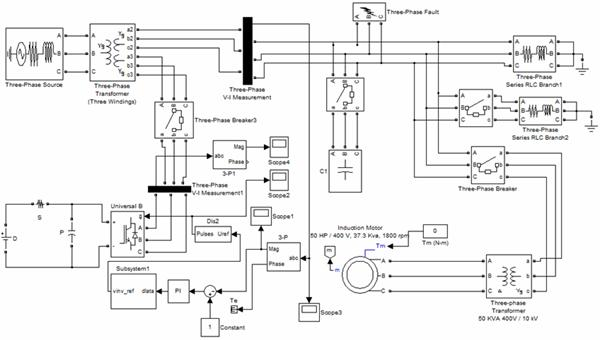Figure 7. Simulation model D-STATCOM connected to distribution system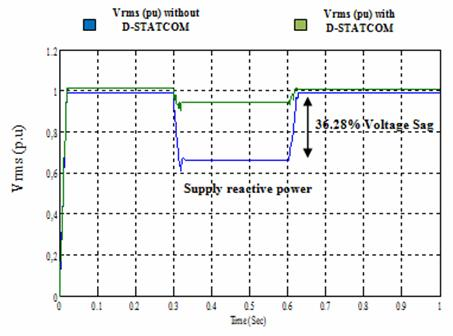Figure 8. Voltage Vrms at load point, with three phase-ground fault without and with D-STATCOM

Case II: A 30μf capacitor bank is connected to the high voltage side of the network, transition time is considered from 0.3 sec to 0.6 sec. The first simulation contains no D-STATCOM, is observed the voltage sag at the load point is 6.67% with respect to the reference voltage as illustrated in Figure 9. The second simulation is carried, but now D-STATCOM is connected to the system, then the voltage sag is mitigated almost completely, and the rms voltage at the sensitive load point is maintained at 99.75% as shown in Figure 9.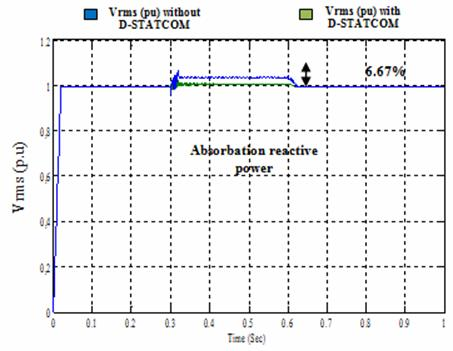Figure 9. Voltage Vrms at load point, with capacitor bank without and with D-STATCOM

Case III: An induction motor is connected to the high voltage side of the network. The first simulation contains no D-STATCOM, is observed the voltage sag at the load point is 17.98% with respect to the reference voltage as illustrated in Fig. 10. The second simulation is carried, but now D-STATCOM is connected to the system, then the voltage sag is mitigated almost completely, and the rms voltage at the sensitive load point is maintained at 99.70% as shown in Figure 10.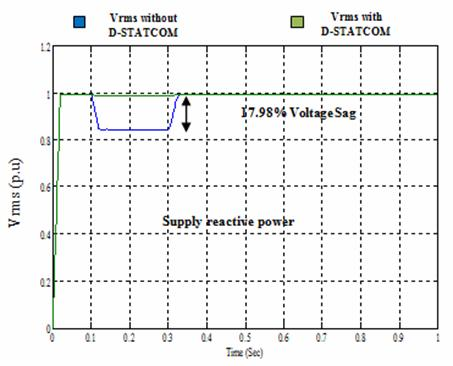Figure 10. Voltage Vrms at load point, with induction motor without and with D-STATCOM

Conclusion

The D-STATCOM has demonstrated excellent performance in every case and it can be concluded that D-STATCOM effectively improves the power quality in distribution networks. It is an attractive technology for future application in the Algerian distribution systems. An actual application of this technology of D-STATCOM is in phase of realization in our laboratory of Intelligent Control and Electrical Power Systems Laboratory ICEPS. Sidi Bel Abbes, Algeria.

This paper shows that D-STATCOM can improve the stability of energy systems especially in critical operating conditions, and unexpected such as short circuits. The work can be expanded in the following area:

1.      Making a comparison between the D-STATCOM based on two and multi-level IGBT voltage source converter.

2.      Dynamic loads can be considered in future work and the effect of D-STATCOM with them can be studied.

3.      Other advanced controllers like fuzzy controller, hysteresis current control, and adaptive fuzzy controller can be employed with D-STATCOM to increase the effectiveness of D-STATCOM in distribution networks.

References

1.      Bhattacharya S., Applications of D-STATCOM Using MATLAB/simulation in power system, Research Journal of Recent Sciences, 2012, 1, p. 430-433.

2.      Sung M., Woo D. W. K., Woo C. L., D S. H., The distribution STATCOM for reducing the effect of voltage sag and swell, IECON’O1: The 27th Annual Conference of the IEEE Industrial Electronics Society Publication, 2001, 2, p. 1132- 1137.

3.      Vishakha T., Design of multi-level converter for power quality enhancement, International Journal of Engineering Research and Reviews, 2014, 2(2), p. 15-21.

4.      Ravi Kumar S.V., Siva Nagaraju S., Simulation of D-STATCOM and DVR in power system, ARPN Journal of Engineering and Applied Sciences, 2007, 2, p. 1819-6608.

5.      Mohammadi M., Akbarinasab M., Voltage sag mitigation with D-STATCOM in distribution systems, Aust. Journal ba. Applied Sciences, 2011, (5), p. 201-207.

6.      Abdelaali A., Contrôle des réseaux electriques par les systèmes FACTS: (Flexible AC transmission systems), Thèse de magister électrotechnique de l’Université de Batna, Algérie, 2009.

7.      Amir H. N., Flexible AC transmission systems -theory, control and simulation of the STATCOM and SSSC, Magister thesis, University of New Brunswick, 2003.

8.      Pradeep K., Niranjan K., Akella A.K., Review of D-STATCOM for stability analysis, IOSR J. Ele. Ele. Engineering (IOSRJEEE), 2012, 1(2), p. 1-9.

9.      John J., Paserba., Masatoshi Takeda., FACTS and custom power equipment for the enhancement of power transmission system performance and power quality, Sym. Spec. Elec. Ope. Expa. Plan, 2000 (VII SEPOPE) Curitiba, p. 21-26.

10.  Ramakrishna V., Madhusudhan R., Power quality improvement of distribution system using distribution static compensator at various fault locations, Sci. eng. res. journal 2014, 12(02), p. 217-224.

11.  Hariyani M., Voltage stability with the help of D-STATCOM, Nat. Conf. Rec. Tren. Eng. Tech., B.V.M. Engineering College, V.V. Nagar, Gujarat, India, 13-14 May, 2011.

12.  Mithilesh K. K., Srivastava S. K., Enhancement in voltage stability and reactive power compensation using D-Statcom, Int. J. Eng. Res. Tech. (IJERT), 2012, 1(7), . ISSN: 2278-0181

13.  Tiwari H.P., Gupta S. K., DVR based on fuel cell: an innovative back-up system, International Journal of Environmental Sci. Dev, 2010, 1(1), p. 73-78, ISSN: 2010-0264.

14.  Gargeya M. A., A new proposal for mitigation of power quality problems using D-STATCOM, International Journal of Scientific Eng. Tech, 2013, 2(5), p. 317-321.